JEE  >  JEE Main Previous Year Questions (2016- 2023): Equilibrium

# JEE Main Previous Year Questions (2016- 2023): Equilibrium - Chemistry for JEE Main & Advanced

Q.1. The ammonia (NH3) released on quantitative reaction of 0.6 g urea (NH2CONH2) with sodium hydroxide (NaOH) can be neutralized by    (2020)
(1) 200 mL of 0.4 N HCl
(2) 200 mL of 0.2 N HCl
(3) 100 mL of 0.2 N HCl
(4) 100 mL of 0.1 N HCl
Ans.
(3)
From the stoichiometry of the reaction,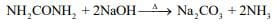1 mol of urea liberates 2 moles of ammonia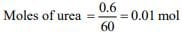Thus, moles of ammonia formed = 2×0.01 = 0.02 mol
Since, 1 mol HCl is required to neutralized 1 mol of ammonia. So, 0.02 moles of HCl is required to neutralized 0.02 moles of ammonia.
From the given concentration of HCl, 100 mL of 0.2 N is equivalent to 0.02 moles of ammonia.
⇒ Moles of HCl = 0.1 × 0.2 = 0.02 moles

Q.2. 3 g of acetic acid is added to 250 mL of 0.1 M HCl and the solution made up to 500 mL. To 20 mL of this solution 1/2mL of 5 M NaOH is added. The pH of the solution is ______.
[Given: pKa of acetic acid = 4.75, molar mass of acetic acid = 60 g mol–1, log 3 = 0.4771]
Neglect any changes in volume.    (2020)
Ans.
(5.23)
Millimoles of AcOH in 20 mL solution = 2
Millimoles of 0.05 M HCl in 20 mL solution = 1
Millimoles of 0.5 mL of 5 M NaOH in 20 mL solution = 2.5
Thus, remaining millimoles of NaOH react with acetic acid in the solution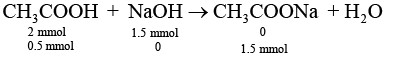From the Henderson Hasselbalch equation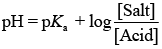...(i)
Substituting the value in Eq. (1), we get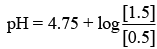⇒ pH = 4.75 + 0.4771   [Given: log 3 = 0.4771]
⇒ pH = 5.23

Q.3. The stoichiometry and solubility product of a salt with the solubility curve given below is, respectively    (2020)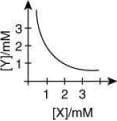(1) X2 Y, 2 x 10-9 M3
(2) XY2, 4 x 10-9 M3
(3) XY2, 1 x 10-9 M3
(4) XY2, 2 x 10-6 M3
Ans.
(2)
From the graph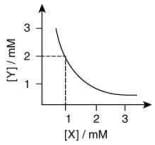Stoichiometry of the compound is XY2
Solubility product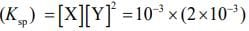= 4 x 10-9 M3

Q.4. Assertion: The pH of water increases with increase in temperature.
Reason: The dissociation of water into H+ and OH is an exothermic reaction.    (2020)
(1) Both assertion and reason are true, and the reason is the correct explanation for the assertion.
(2) Both assertion and reason are false.
(3) Both assertion and reason are true, but the reason is not the correct explanation for the assertion.
(4) Assertion is not true, but reason is true.
Ans.
(2)
With increasing in temperature, the pH of the water will decrease.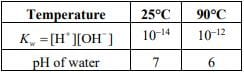The dissociation of water is an endothermic process.

Q.5. The Ksp for the following dissociation is 1.6 × 10−5
PbCl2 (s) ⇌ Pb2+ (aq) 2Cl- (aq)
Which of the following choices is correct for a mixture of 300 mL 0.134 M Pb(NO3)2 and 100 mL 0.4 M NaCl?    (2020)
(1) Not enough data provided
(2) Q < Ksp
(3) Q > Ksp
(4) Q = Ksp
Ans.
(3)
Given,
Ksp (PbCl2) = 1.6 x 10-5
The concentration of Pb2+ from the given solution of Pb(NO3)2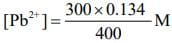The concentration of Cl from the given solution of NaCl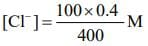Thus, for the reaction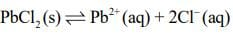The ionic product is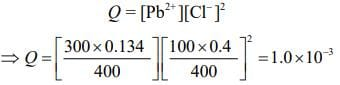Thus, Q > Ksp.

Q.6. The solubility product of Cr(OH)3 at 298 K is 6.0 × 10−31. The concentration of hydroxide ions in a saturated solution of Cr(OH)3 will be    (2020)
(1) (2.22 x 10-31)1/4
(2) (18 x 10-31)1/4
(3) (18 x 10-31)1/2
(4) (4.86 x 10-29)1/4
Ans.
(2)
Given, Ksp = 6.0 x 10-31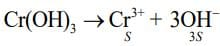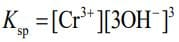⇒ Ksp = S x (3S)3 = 27S4 ...(i)
Substituting the value in Eq. (i), we get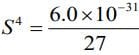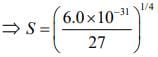...(ii)
The concentration of hydroxide ions in a saturated solution
[OH-] = 3S ...(iii)
Substituting the value from Eq. (2) in Eq. (3), we get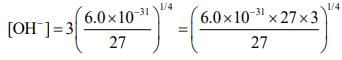⇒ [OH-] = (18.0 x 10-31)1/4

Q.7. In the figure shown below reactant A (represented by square) is in equilibrium with product B (represented by circle). The equilibrium constant is    (2020)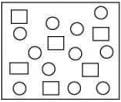(1) 4
(2) 8
(3) 1
(4) 2
Ans.
(4)
From the given figure,
Equilibrium constant (Keq) =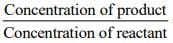= 12/6 = 2

Q.8. The first and second ionization enthalpies of a metal are 4.96 and 4560 kJ mol−1, respectively. How many moles of HCl and H2SO4, respectively, will be needed to react completely with 1 mol of the metal hydroxide?    (2020)
(1) 1 and 1
(2) 2 and 0.5
(3) 1 and 2
(4) 1 and 0.5
Ans.
(4)
Since, the difference between first and second ionization enthalpies is extremely large, so, the metal must an alkali metal. For the completely neutralization of metal hydroxide with HCl and H2SO4.
MOH + HCl → MCl + H2O
2MOH + H2SO4 → M2SO4 + 2H2O
For 1 mol of MOH, 1 mol of HCl and 0.5 mol of H2SO4 is required.

Q.9. Consider the following reversible chemical reactions: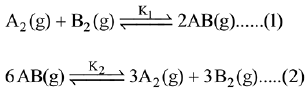The relation between K1 and K2 is:    (2019)
(1) K1K2 = 1/3
(2) K2 = K13
(3) K2 = K1-3
(4) K1K2 = 3
Ans.
(3)
Given: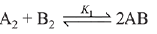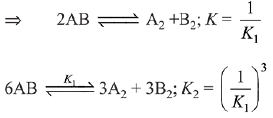The relation between K1 and K2 is K2 = K1-3

Q.10. The values of Kp/Kc for the following reactions at 300 K are, respectively: (At 300 K, RT = 24.62 dm3 atm mol-1)
N2(g) + O2(g) ⇌ 2NO(g)
N2O2(g) ⇌ 2NO2(g)
N2(g) + 3H2(g) ⇌ 2NH3(g)    (2019)
(1) 1, 24.62 dm3 atm mol-1, 606.0 dm6 atm2 mol-2
(2) 1, 24.62 dm3 atm mol-1, 65 x 10-3 dm-6 atm-2 mol2
(3) 1,4.1 x 10-2 dm-3 atm-1 mol, 606 dm6 atm2 mol-2
(4) 24.62 dm3 atm mol-1, 606.0 dm6 atm2 mol-2
1.65 x 10-3 dm-6 atm-2 mol2
Ans.
(2)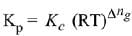Δng = No. of gaseous moles of products - No. of gaseous moles of reactants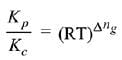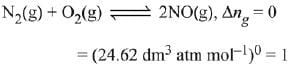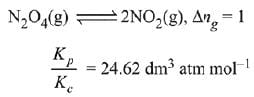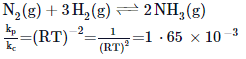Q.11. 5.1 g NH4SH is introduced in 3.0 L evacuated flask at 327°C, 30% of the solid NH4SH decomposed to NH3 and H2S as gases. The Kp of the reaction at 327°C is (R = 0.082 L atm mol-1 K-1, molar mass of S = 32 g mol-1, molar mass of N = 14 g mol-1)    (2019)
(1) 0.242 x 10-4 atm2
(2) 1 x 10-4 atm2
(3) 4.9 x 10-3 atm2
(4) 0.242 atm2
Ans.
(4)
Concerned reaction:
NH4SH → NH3(g) + H2S (g)
Initial moles = 5.1/51 = 0.1 mol
Moles at equillibrium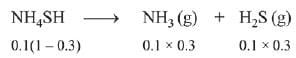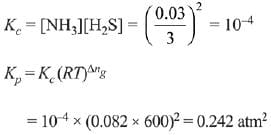Q.12. Consider the reaction
N2(g) + 3H2(g) ⇌ 2NH3(g)
The equilibrium constant of the above reaction is Kp. lf pure ammonia is left to dissociate, the partial pressure of ammonia at equilibrium is given by (Assume that PNH3 <<Ptotal at equilibrium)    (2019)
(1)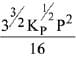(2)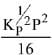(3)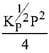(4)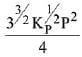Ans.
(1)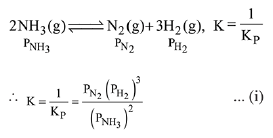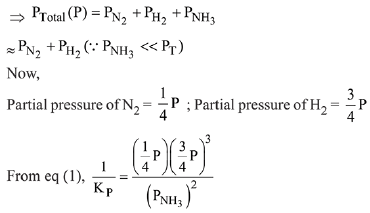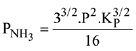Q.13. In a chemical reaction,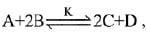the initial concentration of B was 1.5 times of the concentration of A, but the equilibrium concentrations of A and B were found to be equal. The equilibrium constant (K) for the above chemical reaction is:    (2019)
(1) 4
(2) 16
(3) 1/4
(4) 1
Ans.
(1)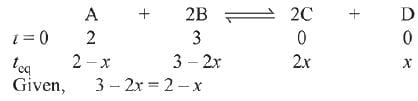⇒ x = 1
∴ [C] = 2, [D] = 1, [A] = 1, [B] = 1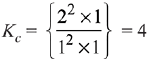Q.14. Two solids dissociate as follows
A(s) → B(g) + C(g); KP1 = x atm2
D(s) → C(g) + E(g); KP2 = y atm2
The total pressure when both the solids dissociate simultaneously is:    (2019)
(1)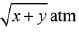(2)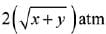(3) (x + y)atm
(4) x2 + y2 atm
Ans.
(2)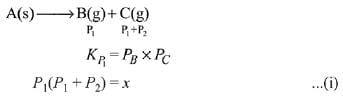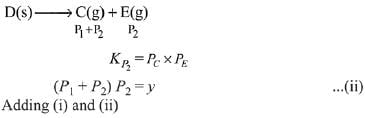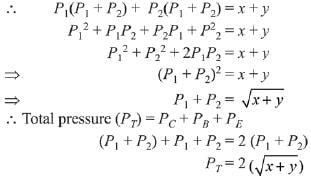Q.15. For the following reactions, equilibrium constants are given: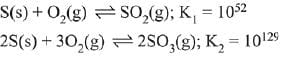The equilibrium constant for the reaction,    (2019)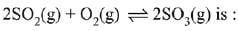(1) 10154
(2) 10181
(3) 1025
(4) 1077
Ans.
(3)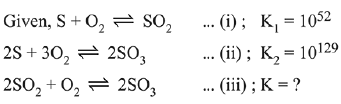To get equation (iii) follow (ii) - 2 (i),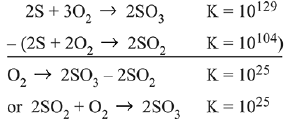Q.16. For the reaction,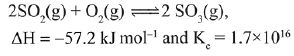Which of the following statement is INCORRECT?    (2019)
(1) The equilibrium constant is large suggestive of reaction going to completion and so no catalyst is required.
(2) The equilibrium will shift in forward direction as the pressure increases.
(3) The equilibrium constant decreases as the temperature increases.
(4) The addition of inert gas at constant volume will not affect the equilibrium constant.
Ans.
(1)
Equilibrium constant has no relation with catalyst. Catalyst only affects the rate of the reaction.
Catalyst V2O5 in the given reaction, is used to speed up the reaction.

Q.17. In which one of the following equilibria, Kp ≠ Kc?    (2019)
(1) 2C(s) + O2(g) ⇌ 2C0(g)

(2) 2HI(g) ⇌ H2(g) + I2(g)
(3) NO2(g) + SO2(g) ⇌ NO(g) + SO3(g)
(4) 2NO(g) ⇌ N2(g) + O2(g)
Ans.
(1)
We know that,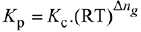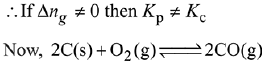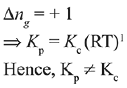Q.18. 20 mL of 0.1 M H2SO4 solution is added to 30 mL of 0.2 M NH4OH solution. The pH of the resultant mixture is: [pKb of NH4OH = 4.7].    (2019)
(1) 522
(2) 9.0
(3) 5.0
(4) 9.4
Ans.
(2)
milli mol of H2SO4 = 20 x 0.1 = 2
milli mol of NH4OH = 30 x 0.2 = 6
H2SO+ 2NH4OH → (NH4)2SO4+ 2H2O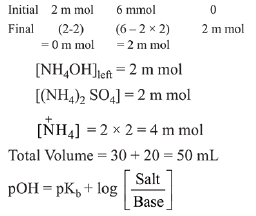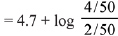= 4.7 + log 2 = 5
pH= 14 - pOH
pH = 14 - 5 = 9

Q.19. For the equilibrium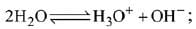the value of ΔG° at 298 K is approximately:    (2019)
(1) 100 kJ mol-1
(2) -80 kJ mol-1
(3) 80 kJ mol-1
(4) -100 kJ mol-1
Ans.
(3)
ΔG = ΔG° + RT In Q
At equilibrium; ΔG = 0 and Q = Keq
⇒ ΔG° = - 2.303 RT log Kw
= -2.303 x 8.314 x 298 x log 10-14
= 79.9 kJ/mol ≈ 80 kJ/mol

Q.20. If Ksp of Ag2CO3 is 8 x 10-12, the molar solubility of Ag2CO3 in 0.1 M AgNO3 is:    (2019)
(1) 8 x 10-12M
(2) 8 x 10-11 M
(3) 8 x 10-10 M
(4) 8 x 10-13 M
Ans.
(3)
As AgNO3 dissociates completely,
therefore in 0.1 M AgNO3 solution, [Ag+] = 0.1 M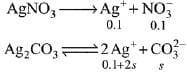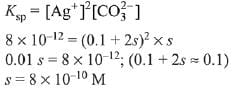Q.21. If solubility product of Zr3(PO4)4 is denoted by Ksp and its molar solubility is denoted by S, then which of the following relation between S and Ksp is correct?    (2019)
(1)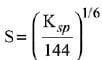(2)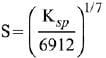(3)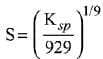(4)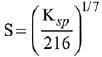Ans.
(2)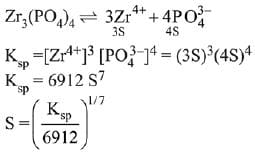Q.22. In an acid base titration, 0.1 M HCI solution was added to the NaOH solution of unknown strength. Which of the following correctly shows the change of pH of the titration mixture in this experiment?    (2019)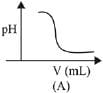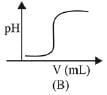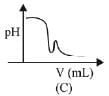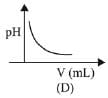(1) (B)
(2) (A)
(3) (C)
(4) (D)
Ans.
(2)
Graph A and B, both represents the titration curve between strong acid and strong base, i.e., HCI and NaOH but, the pH of NaOH is more than 7 and during the titration it decreases, so graph (A) is correct.

Q.23. Consider the following statements    (2019)
(a) The pH of a mixture containing 400 mL of 0.1 M H2SO4 and 400 mL of 0.1 M NaOH will be approximately 1.3.
(b) Ionic product of water is temperature dependent.
(c) A monobasic acid with Ka-10-5 has a pH = 5. The degree of dissociation of this acid is 50%.
(d) The Le Chatelier’s principle is not applicable to common-ion effect.
The correct statements are :
(1) (a) (b) and (d)
(2) (a) (b) and (c)
(3) (b) and (c)
(4) (a) and (b)
Ans.
(2)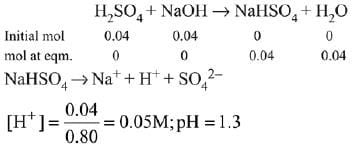(b) Ionic product of water increases with increase in temperature because ionisation of water is endothermic.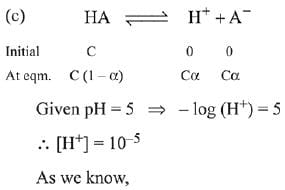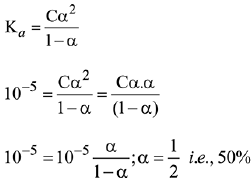Q.24. The pH of a 0.02 M NH4Cl solution will be [given Kb(NH4OH)=10-5 and log 2 = 0.301]    (2019)
(1) 2.65
(2) 4.35
(3) 4.65
(4) 5.35
Ans.
(4)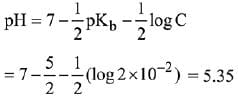pH = 5.35

Q.25. What is the molar solubility of Al(OH)3 in 0.2 M NaOH solution? Given that, solubility product of Al(OH)3 = 2.4 x 10-24:    (2019)
(1) 3 x 10-19
(2) 12 x 10-21
(3) 3 x 10-22
(4) 12 x 10-23
Ans.
(3)
Let the solubility of Al(OH)3 in 0.2M NaOH solution be s.
Then,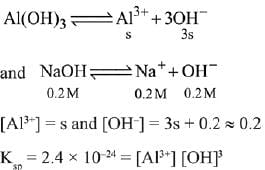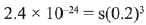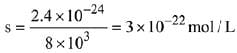Q.26. The molar solubility of Cd(OH)2 is 1.84 x 10-5 M in water. The expected solubility of Cd(OH)2 in a buffer solution of pH= 12 is:    (2019)
(1) 1.84 x 10-9 M
(2)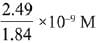(3) 6.23 x 10-11 M
(4) 2.49 x 10-10 M
Ans.
(4)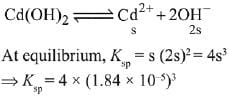Solubility in buffer solution having pH = 12
[OH-] = 10-2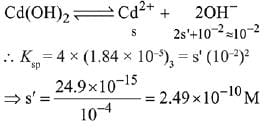Q.27. An aqueous solution contains 0.10 M H2S and 0.20 M HCl. If the equilibrium constants for the format ion of HS- from H2S is 1.0 x 10-7 and that of S2- from HS- ions is 1.2 x 10-13 then the concentration of S2- ions in aqueous solution is:     (2018)
(1) 5 x 10-8
(2) 3 x 10-20
(3) 6 x 10-21
(4) 5 x 10-19
Ans.
(2)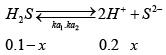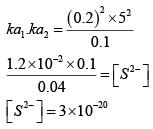Q.28. An aqueous solution contains an unknown concentration of Ba2+. When 50 mL of a 1M solution of Na2SO4 is added, BaSO4 just begins to precipitate. The final volume is 500 mL. The solubility product of BaSO4 is 1 x 10-10. What is the original concentration of Ba2+ ?      (2018)
(1) 5 x 10-9 M
(2) 2 x 10-9 M
(3) 1.1 x 10-9 M
(4) 1.0 x 10-10 M
Ans.
(3)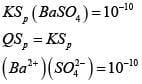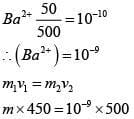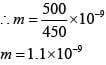Q.29. Which of the following are Lewis acids?      (2018)
(1) PH3 and BCl3
(2) AlCl3 and SiCl4
(3) PH3 and SiCl4
(4) BCl3 and AlCl3
Ans.
(4)
Both BCl3 and AlCl3 are Lewis acids as both ‘B’ and ‘Al’ has vacant p-orbitals. SiCl4 is also a Lewis acid as silicon atom has vacant 3d-orbital.Therefore,both b and d options are possible.

Q.30. Which of the following salts is the most basic in aqueous solution?      (2018)
(1) Al(CN)3
(2) CH3COOK
(3) FeCl3
(4) Pb(CH3COO)2
Ans.
(2)
CH3COOK is a salt of weak acid and strong base. Hydrolysis of potassium acetate gives strong base KOH

Q.31. In which of the following reactions, an increase in the volume of the container will favour the formation of  products?    (2018)
(1) 3O2(g) ⇌ 2O3(g)
(2) 2NO2(g) ⇌ 2NO(g) + O2(g)
(3) 4NH3(g) + 5O2(g) ⇌ 4NO(g) + 6H2O(I)
(4) H2(g) + I2(g) ⇌ 2HI(g)
Ans.
(2)
Volume increases P decreases reaction proceed in that direction where number of gaseous moles increases.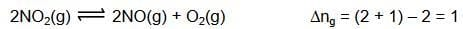Q.32. The minimum volume of water required to dissolve 0.1g lead (II) chloride to get a saturated solution (Ksp of PbCl2 = 3.2 x 10-8; atomic mass of Pb= 207 u) is:    (2018)
(1) 0.18 L
(2) 0.36 L
(3) 17.98 L
(4) 1.798 L
Ans.
(1)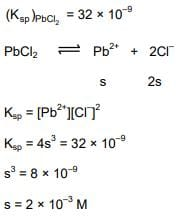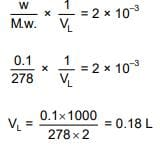Q.33. For which of the following reactions, ΔH is equal to ΔU?    (2018)
(1) N2(g) + 3H2(g) → 2NH3(g)
(2) 2NO2(g) → N2O4(g)
(3) 2SO2(g) + O2(g) → 2SO3(g)
(4) 2HI(g) → H2(g) + I2(g)
Ans. (4)
ΔH = ΔU + ΔngRT.
[If Δng = 0 then ΔH =ΔU]

Q.34. At 320 K, a gas A2 is 20 % dissociated to A(g). The standard free energy change at 320 K and 1 atm in J mol–1 is approximately : (R = 8.314 JK–1 mol–1 ; ln 2 = 0.693 ; ln 3 = 1.098)    (2018)
(1) 1844
(2) 2068
(3) 4281
(4) 4763
Ans. (3)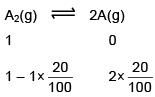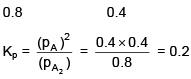ΔGº = –2.303 × 8.314 × 320 log10 0.2 = 4281 J/mole

Q.35. The gas phase reaction 2NO2(g) → N2O4(g) is an exothermic reaction. The decomposition of N2O4, in equilibrium mixture of NO2(g) and N2O4(g), can be increased by:    (2018)
(1) addition of an inert gas at constant pressure.
(2) lowering the temperature
(3) increasing the pressure
(4) addition of an inert gas at constant volume.
Ans. (1)
2NO2(g) → N2O4(g) ΔH = (–)
By addition of an inert gas at constant pressure, volume increases, so reaction moving in backward direction and decomposition of N2O4 increases.

Q.36. pKa of a weak acid (HA) and pKb of a weak base(BOH) are 3.2 and 3.4, respectively, The pH of their salt (AB) solution is    (2017)
(1) 7.2
(2) 6.9
(3) 7.0
(4) 1.0
Ans. 2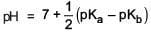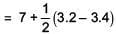= 6.9

Q.37. In the following reactions, ZnO is respectively acting as a/an    (2017)
(a) ZnO + Na2O → Na2ZnO2
(b) ZnO + CO2 → ZnCO3

(1) Base and acid
(2) Base and base
(3) Acid and acid
(4) Acid and base
Ans.  (4)
In (a), ZnO acts as acidic oxide as Na2
O is basic oxide.
In (b), ZnO acts as basic oxide as CO2  is acidic oxide.

Q.38. Addition of sodium hydroxide solution to a weak acid (HA) results in a buffer of pH 6. If ionisation constant of HA is 10–5, the ratio of salt to acid concentration in the buffer solution will be:    (2017)
(1) 10 : 1
(2) 4 : 5
(3) 5 : 4
(4) 1 : 10
Ans.
(1)
HA ⇌ H+ + A-
(Unionised, weak acid and common ion effect)
HA + NaOH → NaA + H2O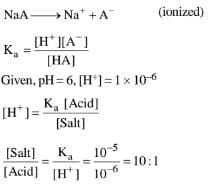Q.39. 50 mL of 0.2 M ammonia solution is treated with 25 mL of 0.2 M HCl. If pKb  of ammonia solution is 4.75, the pH of the mixture will be:    (2017)
(1) 8.25
(2) 9.25
(3) 3.75
(4) 4.75
Ans.
(4)
NH3 + HCI → NH4CI
moles of HCI = 0.2 M x 25 x  10-3 L = 0.005 moles HCI (total consumed)
moles of NH3 = 0.2 M x 50 x 10-3 L = 0.01 moles HCI
excess NH= 0.01 - 0.005 = 0.005 moles
1 mole ammonia = 1 mole NH4CI
0.005 NH3 = 0.005 NH5CI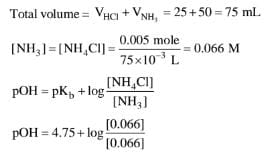pOH = 4.75
pH = 14 - pOH ⇒ pH = 4.75

Q.40. The following reaction occurs in the Blast Furnace where iron ore is reduced to iron metal:
Fe2O3(s) + 3CO(g) ⇌ 2Fe(I) + 3CO2(g)
Using the Le Chatelier’s principle, predict which one of the following will not disturb the equilibrium?    (2017)
(2) Removal of CO2
(4) Removal of CO
Ans.
(3)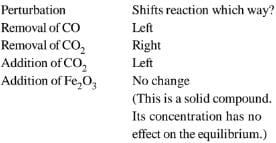Q.41. The equilibrium constant at 298 K for a reaction A + B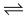C + D is 100. If the initial concentration of all the four species were 1M each, then equilibrium concentration of D (in mol L-1) will be:    (2016)
(1) 0.182

(2) 0.818
(3) 1.818
(4) 1.182
Ans.
(3)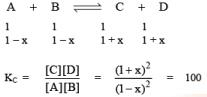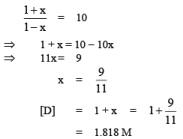Q.42. For the reaction, A(g) + B(g) → C(g) + D(g), ΔH0 and ΔS0 are, respectively, -29.8kJ mol-1 and -0.100 kJ K-1 mol-1 at 298 K. The equilibrium constant for the reaction at 298 K is:    (2016)
(1) 1.0 x 10-10
(2) 10
(3) 1
(4) 1.0 x  1010
Ans.
(3)
ΔG0 = ΔH0 - T.ΔS0
= -29.8 + 298 x (0.1)
= -29.8 + 29.8
∵ ΔG0 = 0
apply relation between ΔG0 & Keq
ΔG0 = -RT ln Keq
ln Keq = 1

Q.43. A solid XY kept in an evacuated sealed container undergoes decomposition to form a mixture of gases X and Y at temperature T. The equilibrium pressure is 10 bar in this vessel. Kp for this reaction is:     (2016)
(1) 25
(2) 5
(3) 10
(4) 100
Ans.
(1)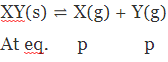Total pressure = 2P = 10 bar
⇒ P = 5
Now, KP = (PX)(PY) = P= 25

The document JEE Main Previous Year Questions (2016- 2023): Equilibrium | Chemistry for JEE Main & Advanced is a part of the JEE Course Chemistry for JEE Main & Advanced.
All you need of JEE at this link: JEE

## Chemistry for JEE Main & Advanced

320 videos|585 docs|368 tests

## Chemistry for JEE Main & Advanced

320 videos|585 docs|368 tests
Signup to see your scores go up within 7 days! Learn & Practice with 1000+ FREE Notes, Videos & Tests.
10M+ students study on EduRev
Track your progress, build streaks, highlight & save important lessons and more!(Scan QR code)
Related Searches

,

,

,

,

,

,

,

,

,

,

,

,

,

,

,

,

,

,

,

,

,

;